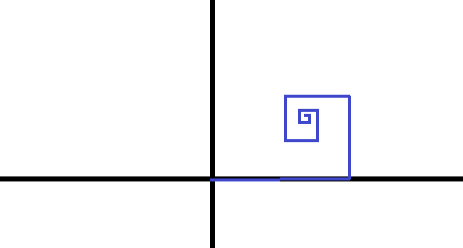# Brilli the bug

Calculus Level 3Brilli the Bug has set out on a journey of infinite steps starting at the origin of the $xy$-plane. It moves in the following manner:

1. After each, $n$th step, it turns $90^\circ$ counter-clockwise
2. Each $n$th step is of length $D_{n}$ where $D_{n}$ is given by $D_{n} = \dfrac{2}{(n+1)^{2}}$ for $n \geq 0$.

If the final displacement of brilli from the starting is given by $\dfrac{1}{\alpha}\sqrt{ \beta G^{\gamma} + \pi^{\eta}}$, find $\alpha +\beta +\gamma +\eta$.

Notation: $G$ denotes the Catalan's constant.

×